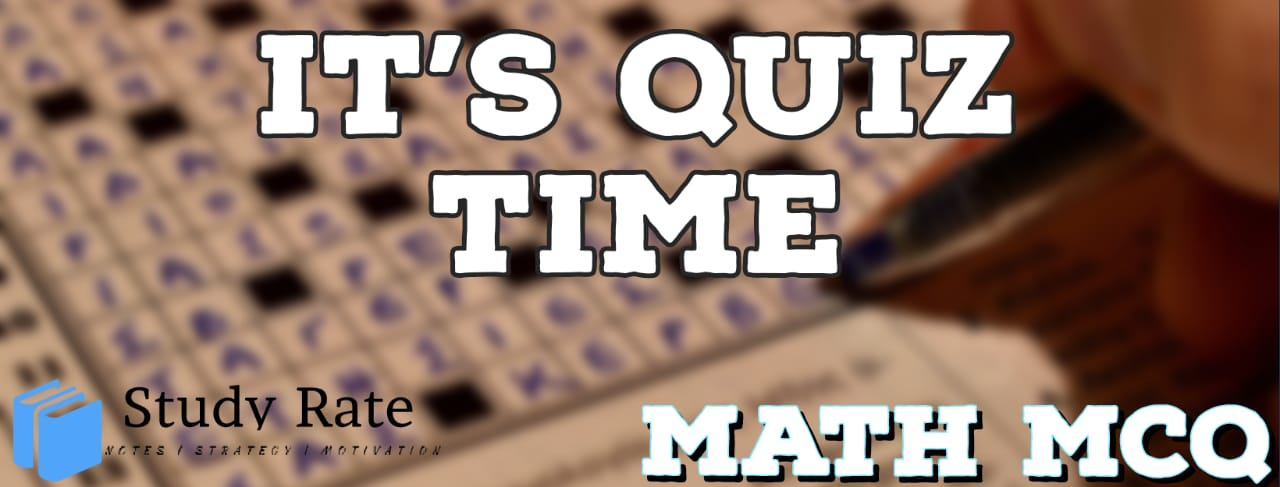## CBSE Class 10 Maths Chapter 15 – Probability MCQ Quiz Online

Free PDF Download of CBSE Class 10 Maths Chapter 15 – Probability Multiple Choice Questions with Answers. MCQ Questions for Class 10 Maths with Answers was Prepared Based on Latest Exam Pattern. Students can solve Class 10 Maths Probability MCQs with Answers to know their preparation level

CBSE Board Class 10 Maths Chapter 15- Probability Objective Questions

1. What is the probability that the minute and hour hands of a clock will form an acute angle at any given time?

2. If P (A) and P (not A) are complementary events and P (A) = 0.15, then P (not A) =?

3. What is the probability of not picking a king if you choose randomly from a pack of 52 cards?

4. 24 cards numbered 1, 2, 3, …., 23, 24 are put in a box and mixed thoroughly. One person draws a card from the box. The probability that the number on the card is divisible by 2 or 3 or both is

5. A single die is rolled. The probability of getting 1 or an even number is

6. From a set of 17 cards, numbered 1, 2,… 17, one card is drawn. What is the probability that the number is a multiple of 3 or 7?

7. What is the probability of getting 53 Mondays in a leap year?

8. A card is drawn from a well shuffled deck of 52 cards. Find the probability of getting a king of red suit

9. A game of chance consists of spinning an arrow which is equally likely to come to rest pointing to one of the number 1,2,3……12 ,then the probability that it will point to an odd number is:

10. A number is selected at random from first 50 natural numbers. Then the probability that it is a multiple of 3 and 4 is: